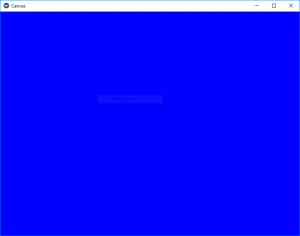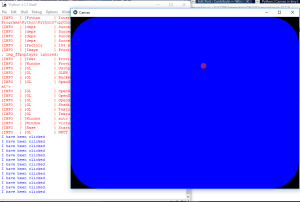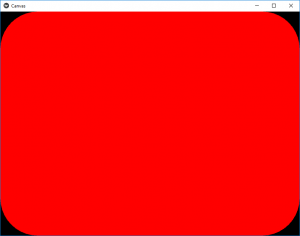Related Articles
Python | Canvas in Kivy using .kv file
• Last Updated : 07 Feb, 2020

Kivy is a platform independent GUI tool in Python. As it can be run on Android, IOS, linux and Windows etc. It is basically used to develop the Android application, but it does not mean that it can not be used on Desktops applications.

## Canvas :

The Canvas is the root object used for drawing by a Widget. A kivy canvas is not the place where you paint.
Each Widget in Kivy already has a Canvas by default. When you create a widget, you can create all the instructions needed for drawing. If self is your current widget. The instructions Color and Rectangle are automatically added to the canvas object and will be used when the window is drawn.

To use Canvas you must have to import:

`from kivy.graphics import Rectangle, Color`
```Basic Approach -
-> import kivy
-> import kivy App
-> import widget
-> import Canvas i.e.:
from kivy.graphics import Rectangle, Color
-> set minimum version(optional)
-> Extend the Widget class
-> Create the App Class
-> create the .kv file:
-> create the canvas
-> return a Widget
-> Run an instance of the class```
Implementation of the Approach:

1) Creating A simple canvas:

main.py file

 `# import kivy module  ` `import` `kivy  ` `   `  `# this restrict the kivy version i.e  ` `# below this kivy version you cannot  ` `# use the app or software  ` `kivy.require(``"1.9.1"``)  ` `   `  `# base Class of your App inherits from the App class.  ` `# app:always refers to the instance of your application  ` `from` `kivy.app ``import` `App  ` ` `  `# A Widget is the base building block ` `# of GUI interfaces in Kivy. ` `# It provides a Canvas that ` `# can be used to draw on screen. ` `from` `kivy.uix.widget ``import` `Widget ` ` `  `# From graphics module we are importing ` `# Rectangle and Color as they are ` `# basic building of canvas. ` `from` `kivy.graphics ``import` `Rectangle, Color ` ` `  ` `  `# class in which we are creating the canvas ` `class` `CanvasWidget(Widget): ` `    ``pass` ` `  `# Create the App Class ` `class` `CanvasApp(App): ` `    ``def` `build(``self``): ` `        ``return` `CanvasWidget() ` ` `  `# run the App ` `CanvasApp().run() `

Canvas.kv file:

 `# .kv file of canvas ` ` `  ` ` ` `  `    ``# creating canvas ` `    ``canvas: ` `        ``Color: ` `            ``rgba: ``0``, ``0``, ``1``, ``1`    `# Blue ` ` `  `        ``# size and position of Canvas ` `        ``Rectangle: ` `            ``pos: ``self``.pos ` `            ``size: ``self``.size `

Output:Now How can we change the color of canvas by any action so below is the example of in which by clicking the color of screen changes.

main.py file:

 `# import kivy module  ` `import` `kivy  ` `   `  `# this restrict the kivy version i.e  ` `# below this kivy version you cannot  ` `# use the app or software  ` `kivy.require(``"1.9.1"``)  ` `   `  `# base Class of your App inherits from the App class.  ` `# app:always refers to the instance of your application  ` `from` `kivy.app ``import` `App  ` ` `  `# From graphics module we are importing ` `# Rectangle and Color as they are ` `# basic building of canvas. ` `from` `kivy.graphics ``import` `Rectangle, Color ` ` `  `# The ButtonBehavior mixin class provides Button behavior. ` `from` `kivy.uix.button ``import` `ButtonBehavior ` ` `  `# The Label widget is for rendering text.  ` `from` `kivy.uix.label ``import` `Label ` ` `  `# class in which we are creating the canvas ` `class` `CanvasWidget(ButtonBehavior, Label): ` `    ``pass` ` `  `# Create the App Class ` `class` `CanvasApp(App): ` `    ``def` `build(``self``): ` `        ``return` `CanvasWidget() ` ` `  `# run the App ` `CanvasApp().run() `

.kv file:

 `# .kv file of canvas ` ` `  ` ` ` `  `    ``# Creating Canvas ` `    ``canvas: ` ` `  `        ``# Color is blue if button is pressed, ` `        ``# otherwise color is red ` `        ``Color:  ` `            ``rgb: (``1``, ``0``, ``0``, ``1``) ``if` `self``.state ``=``=` `'normal'` `else` `(``0``, ``0``, ``1``, ``1``) ` `     `  `        ``# Rounded rectangle canvas ` `        ``RoundedRectangle: ` `            ``size: ``self``.size ` `            ``pos: ``self``.pos ` `             `  `            ``# Play with these if you want smooth corners for your button ` `            ``radius: ``100``, ``100``, ``100``, ``100` `         `  ` `  `    ``# Print the text when touched or button pressed     ` `    ``on_release: ` `        ``print``(``"I have been clicked"``) `

Output:Note:
Kivy drawing instructions are not automatically relative to the widgets position or size. You therefore you need to consider these factors when drawing. In order to make your drawing instructions relative to the widget, the instructions need either to be declared in the KvLang or bound to pos and size changes.

Attention geek! Strengthen your foundations with the Python Programming Foundation Course and learn the basics.

To begin with, your interview preparations Enhance your Data Structures concepts with the Python DS Course.

My Personal Notes arrow_drop_up
Recommended Articles
Page :